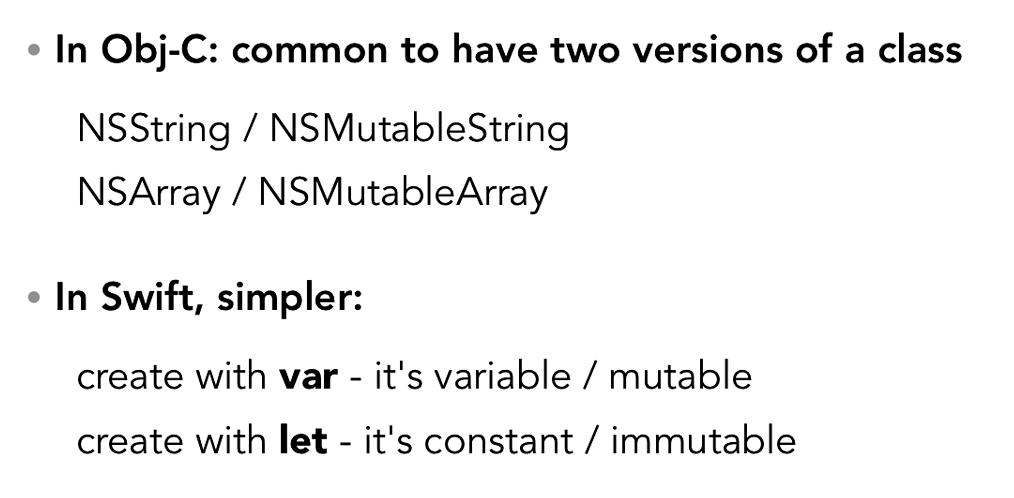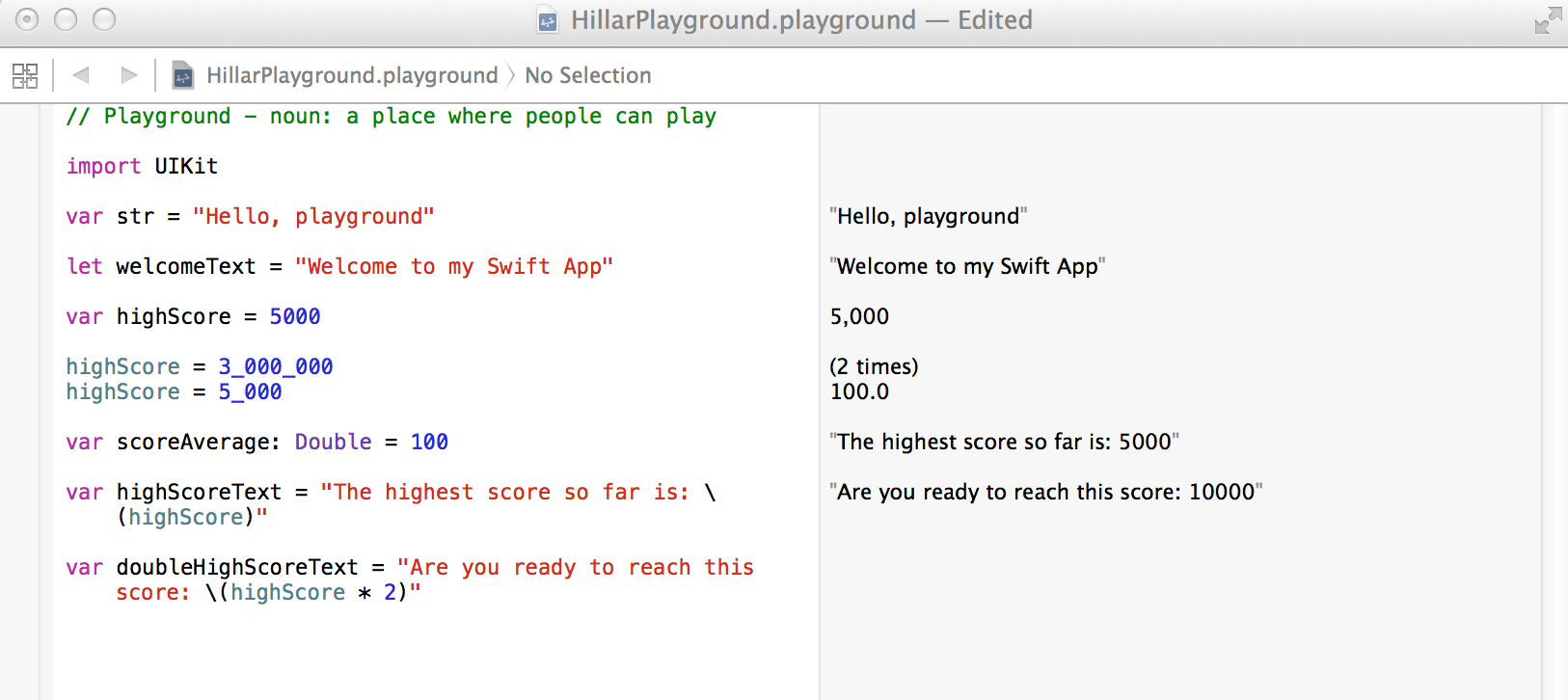## Swift – Constants & Literals

### 1. Constant

Constants values in swift language refer to fixed values that a program may not alter during its run time. Constants can be of any of different basic data types like an integer, float, character, string literal, enumeration etc.

Constants values are nothing but variable whose value cannot be modified after definition.Constants Declaration

To declare a constant variable we have to use let keyword::

Syntax –

let Constant_Variable_Name = <Initial Value>

Example : –

Import Cocoa

let constantValue = 72

print(constantValue)

Running above program using the playground, we get the result as follows::

72

### 2. Type Annotaion

Type Annotaion is helpful to show the value that a constant can store.

Syntax :-

var constantName:<data type> = <Initial VAlue>

Example :-

Import Cocoa

var constantA:Int = 45

var constant:Float = 34.44

print(constantA)

print(constantB)

Running above program using the playground, we get the result as follows::

45

34.44

3. Naming Constant

To name a constant value we can use letters, digits, underscore, character. It must start with letter or an underscore. Upper and lower case letter are different in swift as it a case-sensitive programming language.

Constant and variable names must not include whitespace characters,  mathematical symbols, arrows, private-use (or invalid) Unicode code points, or line- and box-drawing characters. Also cannot begin with a number, although numbers may be included elsewhere within the name of the constant and variable.

Example:-

Import Cocoa

let _constA = “Hello Brother”

let constant_12 = 324

print(_constA)

print(constant_12)

Running above program using the playground, we get result as follows::

Hello Brother

324

### 4. Printing Constant

Printing Constant in swift can be done using print function.

We can print constant value by wrapping the constant_name in parentheses and escape it with a backslash before opening parentheses.

Example : –

Import Cocoa

let constantA = “Diamond”

let constantB =  “10”

Print(“Cost of |(constantA) is more than |(constantB) thousand”)

Running above program using the playground, we get result as follows ::

Cost of Diamond is more than 10 thousandComments are used to include nonexecutable text in our code, as a note or reminder to for developer. Comments are ignored by the Swift compiler when your code is compiled at compile time.

There are different types of comments::

Single-line comments begin with double forward-slashes.

// This is a comment.

Multiline comments begin with forward-slash followed by an asterisk  (/*) and at the end an asterisk followed by a forward-slash  (*/):

/* This is also a comment

but is written over multiple lines. */

### Literals

A literal represent the source code value of an integer, floating-point number, or string type.

Following are some Examples ::

47         // Integer Literals

3.1546    // Floating-Point Literals

“Bitware Technologies”   // String Literals

### 1. Integer Literals

An integer literals can be represented by decimal, binary, octal, or hexadecimal constant. Ocatl literals begin with 0o, binary literals begin with 0b, and hexadecimal literals begin with 0x.

Examples of Integer Literals : –

let decimalInteger = 16           // 16 in decimal notation

let binaryInteger = 0b10000       // 16 in binary notation

let octalInteger = 0o20           // 16 in octal notation

### 2. Floating-point Literals

Floating-point literal consist of an integer part, a decimal point, a fractional part, with or without an exponent part. Foating point literals can be represented  either in decimal form or hexadecimal form.

Decimal floating-point literals consist of a decimal digits followed by either a decimal fraction, a decimal exponent, or both.

Hexadecimal floating-point literals  consist of a 0x prefix, followed by an optional hexadecimal fraction value, followed by a hexadecimal exponent(e).

Examples  of  Floating point Literals : –

let decimalDouble     = 12.18753            let exponentDouble    = 1.21875e3       let hexadecimalDouble = 0xC.3p0

### 3. String Literals

A string literal is represented by double quotes with sequence of characters in it.

String literals cannot contain an unescaped double quote (“), an unescaped backslash (\), a carriage return, or a line feed. Special characters can be included in string literals using the following escape sequences −

 Escape sequence Meaning \0 Null Character \\ \character \b Backspace \f Form feed \n Newline \r Carriage return \t Horizontal tab \v Vertical tab \’ Single Quote \” Double Quote \000 Octal number of one to three digits \xhh… Hexadecimal number of one or more digits

Example  for string literals : −

import Cocoa

let stringLL = “Bitware\tTechnologies\n\nMachine\’Testing\'”

print(stringLL)

Run program using playground, we get the below result

Bitware Technologies

Machine’Testing’

### 4. Boolean Literals

Boolean Literals represent three value. All are part of swift Keywords.

• Value true is represented by true.
• Value false is represented by
• No value is represented by nil.

### Dinesh ChoudharyiOS Developer with 2+ years of experience.